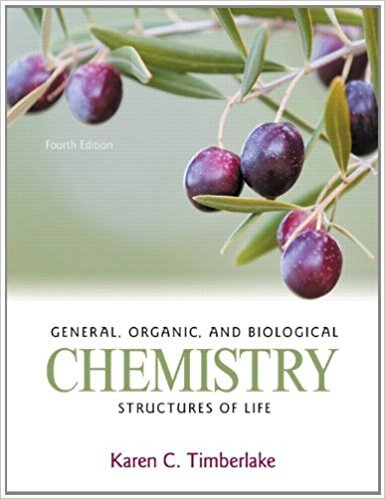×

# Solutions for Chapter 24: General, Organic, and Biological Chemistry: Structures of Life 4th Edition## Full solutions for General, Organic, and Biological Chemistry: Structures of Life | 4th Edition

ISBN: 9780321750891Solutions for Chapter 24

Solutions for Chapter 24
4 5 0 401 Reviews
12
2
##### ISBN: 9780321750891

This expansive textbook survival guide covers the following chapters and their solutions. General, Organic, and Biological Chemistry: Structures of Life was written by and is associated to the ISBN: 9780321750891. Chapter 24 includes 98 full step-by-step solutions. This textbook survival guide was created for the textbook: General, Organic, and Biological Chemistry: Structures of Life, edition: 4. Since 98 problems in chapter 24 have been answered, more than 63656 students have viewed full step-by-step solutions from this chapter.

Key Chemistry Terms and definitions covered in this textbook
• amino acids.

A compound that contains at least one amino group and at least one carboxyl group. (25.3)

• amorphous solid.

A solid that lacks a regular three-dimensional arrangement of atoms or molecules. (11.7)

• amphoteric oxides and hydroxides

Oxides and hydroxides that are only slightly soluble in water but that dissolve in either acidic or basic solutions. (Section 17.5)

• Autoxidation

Air oxidation of materials such as unsaturated fatty acids.

• Carbene

A neutral molecule that contains a carbon atom surrounded by only six valence electrons (R2C:).

• chelating agent.

A substance that forms complex ions with metal ions in solution. (23.3)

• chemical formula

A notation that uses chemical symbols with numerical subscripts to convey the relative proportions of atoms of the different elements in a substance. (Section 2.6)

• dimensional analysis

A method of problem solving in which units are carried through all calculations. Dimensional analysis ensures that the final answer of a calculation has the desired units. (Section 1.6)

• Henderson–Hasselbalch equation

The relationship among the pH, pKa, and the concentrations of acid and conjugate base in an aqueous solution: pH = pKa + log 3base4 3acid4. (Section 17.2)

• Homotopic groups

Atoms or groups on an atom that give an achiral molecule when one of the groups is replaced by another group. The hydrogens of the CH2 group of propane, for example, are homotopic. Replacing either one of them with deuterium gives 2-deuteropropane, which is achiral. Homotopic groups have identical chemical shifts under all conditions

• norbornane

The common name for bicyclo[2.2.1]heptane.

• polyether

A compound containing several ether groups.

• Quantized

Having specifi c values for energy and momentum

• Reduction

The gain of electrons. Alternatively, either the gain of hydrogen, loss of oxygen, or both

• Robinson annulation

The combination of a Michael addition followed by an aldol condensation to form a ring.

• sigmatropic rearrangements

A pericyclic reaction in which one s bond is formed at the expense of another.

• sp-hybridized

Atomic orbitals that are achieved by mathematically averaging one s orbital with only one p orbital to form two hybridized atomic orbitals.

• spin-pairing energy

The energy required to pair an electron with another electron occupying an orbital. (Section 23.6)

An addition reaction in which two groups are added to the same face of a p bond.

• Z (Section 5.2C)

From the German, zusammen, meaning opposite. Specifi es that groups of higher priority on the carbons of a double bond are on the same side

×